Home > A2C > Chapter 8 > Lesson 8.2.2 > Problem8-127

8-127.
1. Find an equation for each graph below. Homework Help ✎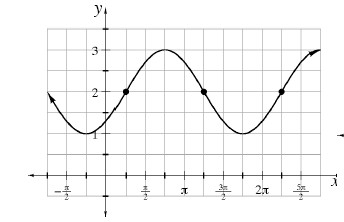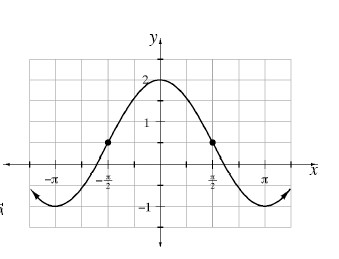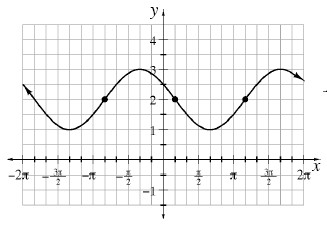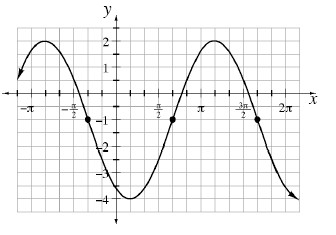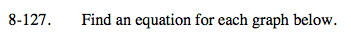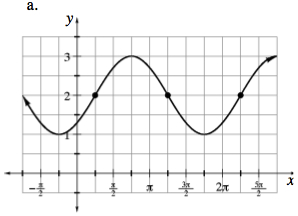Use the general equation y = a · sin b(xh) + k.
Determine the value for each of the four parameters.

First choose a function (sine or cosine) by identifying a convenient locator point.
In this case, we will use:

$\left(\frac{\pi}{4}, 2\right)$

Since our point is on the midline, we will use the cosine function.
The x-value of the point also represents the horizontal shift:

$h=\frac{\pi}{4}$

Next find k, the vertical shift.
The graph y = sin x has a midline at y = 0 (the x-axis).
The parameter k is the distance that midline is shifted vertically.
In this case k = 2.

The amplitude (a) is the distance from the midline (k) to the highest point.
In this case k = 2 and the highest point is 3, so a = 1.
Since the graph increases from the locator point in the same way y = sin x does, a is positive.

You will learn more about the parameter b in future lessons.
For now, know that b = 1 since the distance from the beginning to the end of one cycle is 2π.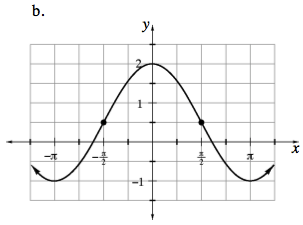See part (a).
Pay careful attention to the scale on the y-axis when determining a and k.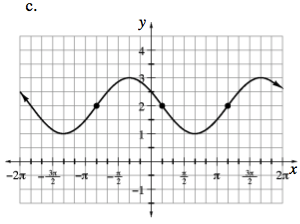$\text{If you choose } \left(\frac{\pi}{6},0\right) \text{ as your locator point,}$

notice the first cycle of the graph is an inverted sine curve, so the a value will be negative.

See part (a).

$y=\text{-sin}\left(x-\frac{\pi}{6}\right)+2\text{ or }y=\text{sin}\left(x+\frac{5\pi}{6}\right) +2$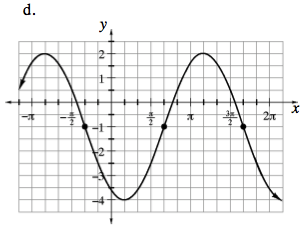See parts (a) and (c).